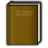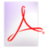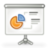### The Disjoint Paths Problems: an annotated tableau## Preliminary

This page is the online version of the tableau , a joint work with András Sebő, that appeared in the book Research Trends in Combinatorial Optimization . It is an attempt to capture some of the most important variants of the disjoint paths problems, as well as pointing out some open problems. We only considered the decision versions of the problems. We believe that an optimization version of the tableau, including approximability, would also be necessary.

Despite all our care in making this tableau, it is still possible that we missed some known results. If you find any error or omission, or have any constructive remark, it would be most helpful to send me an email.

The main definitions concerning disjoint paths problems, and the notations can be found on this page.

The tableau is divided into three parts:

Each part is then further divided between the undirected, directed and directed acyclic cases, and depending on the nature of $H$ and the quantity of request. You will find a bibliography at the foot of this page.

## $G$ is arbitrary

directed directed acyclic undirected
$E$-d $V$-d $E$-d $V$-d $E$-d $V$-d
$|H|$ $r$ $gen$ $Eul$ $gen$ $Eul$ $gen$ $Eul$
arb. bin NPC NPC NPC NPC NPC NPC NPC NPC NPC
un NPC NPC NPC NPC NPC NPC
fix bin NPC NPC NPC NPC NPC P NPC NPC P
un NPC NPC NPC NPC NPC
fix NPC P P
2 bin NPC NPC P P NPC P P
un NPC P P NPC P
fix NPC P P P NPC P
2 P P P P P

A remarkable fact is that here only one cell is open, namely the arc-disjoint paths problem for Eulerian instances with a fixed total request and $|E(H)| \geq 3$. The simplest version is actually in P, where one wants to decide the existence of three disjoint paths, as asserted by the Theorem 8 of Ibaraki and Poljak stated below. If the total request is $4$ the problem is open.

The main theorems, in an arbitrary order:

Theorem 1: (Even , Itai, Shamir ) The edge-disjoint paths problem in undirected graphs and the arc-disjoint paths problem in directed acyclic graphs is NP-complete,, even if $r(E(H)) = 3$.

Theorem 2: (Fortune , Hopcroft, Wyllie ) The vertex-disjoint paths problem is NP-complete, even if $r(E(H)) = 2$.

Theorem 3: (Vygen ) The integer multicommodity flow problem is NP-complete even if $G$ is an acyclic directed graph, $r+c$ is Eulerian and $|E(H)| = 3$.

Theorem 4: (Frank ) The integer multicommodity flow problem in Eulerian digraphs with $|E(H)| = 2$ is solvable in polynomial-time. The cut condition is sufficient for the existence of a solution.

Theorem 5: (Fortune , Hopcroft, Wyllie ) The arc-disjoint paths problem in directed acyclic graph with $r(E(H))$ bounded is solvable in polynomial-time.

Theorem 6: (Lomonosov ) The undirected integer multicommodity flow problem is solvable in polynomial-time in Eulerian instances when $H$ is the union of two stars, or $K_4$, or $C_5$. In these cases, the cut condition is sufficient.

A weaker version of the previous theorem was proved by Rothschild and Whinston  for the case when $H$ is the union of two stars.

Theorem 7: (Robertson , Seymour ) The vertex-disjoint paths problem in undirected graphs with $r(E(H))$ bounded is solvable in polynomial-time. By usual reduction, under the same condition the edge-disjoint paths problem is also in P.

Theorem 8: (Ibaraki , Poljak ) The arc-disjoint paths problem in Eulerian instances with $r(E(H)) = 3$ is in P.

The following powerful lemma from Vygen allows to extend some results from DAG to undirected graphs when the Eulerian condition is assumed. It is used several times in the tableau.

Lemma 9: (Vygen ) Let $G,H,r,c$ be an instance of the arc-disjoint paths problem with $G+H$ Eulerian and $G$ acyclic. Let $G',H'$ be obtained by neglecting the orientation of $G,H$ respectively. There is a solution to the arc-disjoint paths problem in $G,H,r,c$ iff there is a solution to the edge-disjoint paths problem in $G',H',r,c$.

## $G$ is planar

directed directed acyclic undirected
$E$-d $V$-d $E$-d $V$-d $E$-d $V$-d
$|H|$ $r$ $gen$ $Eul$ $gen$ $Eul$ $gen$ $Eul$
arb. bin NPC NPC NPC NPC NPC NPC NPC NPC NPC
un NPC NPC NPC NPC NPC NPC
fix bin NPC ??? P NPC ??? P NPC ??? P
un NPC ??? NPC P NPC ???
fix ??? ??? P P P P
2 bin NPC P P NPC P P NPC P P
un NPC P NPC P NPC P
fix ??? P P P P P
2 ??? P P P P P

Theorem 10: (Marx ) The arc-disjoint paths problem is NP-complete on Eulerian instances even if $G$ is planar and acyclic. The edge-disjoint paths problem is NP-complete on Eulerian instances even if $G$ is undirected and planar.

Theorem 11: (Naves ) The integer multicommodity flow problem is NP-complete even with one of the following restrictions:

• $G$ is a planar undirected graph, $H$ has only two edges with endpoints on the same face of $G$,
• $G$ is a directed graph, $G+H$ is planar and $|V(H)| = 2$,
• $G$ is a directed acyclic planar graph, $H$ has only two arcs with endpoints on the same face of $G$.

Dirk Müller  proved in 2006 the weaker case when $G$ is directed and planar and $|V(H)| = 2$.

Theorem 12: (Schrijver ) The vertex-disjoint paths problem in planar digraphs with $|E(H)|$ bounded is solvable in polynomial-time.

Theorem 13: (Naves ) The edge-disjoint paths problem is solvable in polynomial-time on Eulerian instances when $G$ is a directed acyclic planar graph and $|E(H)|$ is bounded.

## $G+H$ is planar

directed directed acyclic undirected
$E$-d $V$-d $E$-d $V$-d $E$-d $V$-d
$|H|$ $r$ $gen$ $Eul$ $gen$ $Eul$ $gen$ $Eul$
arb. bin NPC ??? NPC P P NPC NPC P NPC
un NPC ??? P P NPC P
fix bin NPC ??? P P P P P P P
un NPC ??? P P P P
fix ??? ??? P P P P
2 bin NPC P P P P P P P P
un NPC P P P P P
fix ??? P P P P P
2 ??? P P P P P

Theorem 14: (Middendorf , Pfeiffer ) The edge-disjoint paths problem is NP-complete even if $G+H$ is planar.

From the previous theorem, we derived a directed version:

Theorem 15: (Naves , Sebő ) The vertex-disjoint paths problem in acyclic digraphs with $G+H$ planar is NP-complete.

Theorem 16: (Lucchesi , Younger ) The integer multicommodity flow problem is in P when restricted to instances where $G$ is a directed acyclic graph and $G+H$ is planar.

Theorem 17: (Seymour ) The integer multicommodity flow problem is in P when restricted to Eulerian instances where $G$ is undirected and $G+H$ does not contain a $K_5$-minor (in particular when $G+H$ is planar).

Theorem 18: (Sebő ) The integer multicommodity flow problem is in P when $G$ is undirected, $G+H$ is planar and $|E(H)|$ is bounded.

Back to the top.

## Bibliography

1.Paths, Flows, and VLSI-Layout.
Springer-Verlag New York, Inc. Secaucus, NJ, USA, (1990).
2.Research Trends in Combinatorial Optimization.
Springer Verlag, (2008).
3.Marie-Christine Costa, Lucas Létocart, Frédéric Roupin: Minimal multicut and maximal integer multiflow: a survey.
European Journal of Operational Research, 162, 55 -- 69.
Elsevier, (2005).
4.Shimon Even, Alon Itai, Adi Shamir: On the Complexity of Timetable and Multicommodity Flow Problems.
SIAM Journal on Computing, 5, 691 -- 703.
(1976).
5.Steven Fortune, John E. Hopcroft, James Wyllie: The Directed Subgraph Homeomorphism Problem.
TCS: Theoretical Computer Science, 10.
(1980).
6.András Frank: Edge-disjoint paths in planar graphs.
Journal of combinatorial theory. Series B, 39, 164 -- 178.
7.András Frank: On Connectivity Properties of Eulerian Digraphs.
Annals of Discrete Mathematics, 41, 179 -- 194.
(1989).
8.András Frank: Packing paths in planar graphs.
Combinatorica, 10, 325 -- 331.
9.András Frank: Packing paths, circuits and flows.
In: Paths, Flows and VLSI Layouts. Editors:Bernhard Korte, László Lovász, Hans Jürgen Prőmel, Alexander Schrijver., 47 -- 100.
Springer, (1990).
10.András Frank, Éva Tardos, András Sebő: Covering directed and odd cuts.
Mathematical Programming study 22, 99 -- 112.
North-Holland, (1984).
11.Jim Geelen, Bertrand Guenin: Packing odd circuits in Eulerian graphs.
Journal of Combinatorial Theory, Series B, 86, 280 -- 295.
Elsevier, (2002).
12.Toshihide Ibaraki, Svatopluk Poljak: Weak Three-Linking in Eulerian Digraphs.
SIAM journal on Discrete Mathematics, 4, 84 -- 98.
(1991).
13.Richard M. Karp: On the computational complexity of combinatorial problems.
Networks, 5, 45 -- 68.
(1975).
14.Mark R. Kramer, Jan van Leeuwen: The complexity of wire-routing and finding minimum area layouts for arbitrary VLSI circuits.
Advances in computing research, 2, 129 -- 146.
(1984).
15.Michael Lomonosov: Combinatorial approaches to multiflow problems.
Discrete Applied Mathematics, 11, 1 -- 93.
(1985).
16.Michael Lomonosov, András Sebő: On the geodesic-structure of graphs: a polyhedral approach to metric decomposition.
In: Proceedings of the 3rd International Conference on Integer Programming and Combinatorial Optimization, IPCO'93 (Erice, Sicily, April 29 - May 1, 1993). Editors:Giovanni Rinaldi, Lawrence A. Wolsey., 221 -- 234.
CORE, Mathematical Programming Society, (1993).
17.Cláudio Leonardo Lucchesi, Daniel H. Younger: A minimax theorem for directed graphs.
J. London Math. Soc, 17, 369 -- 374.
(1978).
18.James F. Lynch: The equivalence of theorem proving and the interconnection problem.
ACM SIGDA Newsletter, 5, 31 -- 36.
ACM New York, NY, USA, (1975).
19.Dániel Marx: Eulerian disjoint paths problem in grid graphs is NP-complete.
Discrete Applied Mathematics, 143, 336 -- 341.
Elsevier, (2004).
20.Matthias Middendorf, Frank Pfeiffer: On the complexity of the disjoint paths problem.
Combinatorica, 13, 97 -- 107.
Springer, (1993).
21.Dirk Müller: On the complexity of the planar directed edge-disjoint paths problem.
Mathematical Programming, 105, 275 -- 288.
Springer, (2006).
22.Hiroshi Nagamochi, Toshihide Ibaraki: Multicommodity flows in certain planar directed networks.
Discrete Appl. Math., 27, 125 -- 145.
(1990).
23.Guyslain Naves: The hardness of routing two pairs on one face.
Mathematical Programming, 131, 49 -- 69.
Springer, (2012).24.Guyslain Naves: On disjoint paths in acyclic planar graphs.
Submitted, (2010).25.Guyslain Naves, András Sebő: Multiflow Feasibility: an annotated tableau.
In: Research Trends in Combinatorial Optimization. Editors:William J. Cook, László Lovász, Jens Vygen., 261 -- 283.
Springer, (2008).26.Haruko Okamura, Paul D. Seymour: Multicommodity flows in planar graphs.
J. Combinat. Theory, Ser. B, 31, 75 -- 81.
(1981).
27.Kenji Onaga, O. Kakusho: On feasibility conditions of multicommodity flows in networks.
IEEE Transactions on Circuit Theory, 18, 425 -- 429.
(1971).
28.Frank Pfeiffer: Zur Komplexitat des Disjunkte-Wege-Problems.
PhD. thesis, Universität Bonn, (1990).
29.Neil Robertson, Paul D. Seymour: Graph minors. XIII. The disjoint paths problem.
Journal of Combinatorial Theory, Series B, 63, 65 -- 110.
Elsevier, (1995).
30.Bruce Rothschild, Andrew Whinston: Feasibility of two commodity network flows.
Operations Research, 1121 -- 1129.
Operations Research Society of America, (1966).
31.Alexander Schrijver: The Klein bottle and multicommodity flows.
Combinatorica, 9, 375 -- 384.
Springer, (1989).
32.Alexander Schrijver: Applications of polyhedral combinatorics to multicommodity flows and compact surfaces.
In: Polyhedral Combinatorics: Proceedings of a DIMACS Workshop: June 12-16, 1989. , 119 -- 137.
(1990).
33.Alexander Schrijver: Homotopic routing methods.
Paths, Flows, and VLSI-Layout, 329 -- 371.
Springer Verlag, (1990).
34.Alexander Schrijver: Complexity of Disjoint Paths Problems in Planar Graphs.
In: Proceedings of the First Annual European Symposium on Algorithms. , 357 -- 359.
(1993).
35.Alexander Schrijver: Finding $k$ Disjoint Paths in a Directed Planar Graph.
SIAM Journal on Computing, 23, 780 -- 788.
(1994).
36.Alexander Schrijver: Combinatorial optimization: polyhedra and efficiency.
Springer, (2003).
37.Alexander Schrijver: Theory of linear and integer programming.
Wiley, (1986).
38.Werner Schwärzler: On the complexity of the planar edge-disjoint paths problem with terminals on the outer boundary.
Combinatorica, 29, 121 -- 126.
Springer, (2009).
39.András Sebő: Integer plane multiflows with a fixed number of demands.
Journal of Combinatorial Theory Series B, 59, 163 -- 171.
Academic Press, Inc. Orlando, FL, USA, (1993).
40.Paul D. Seymour: Decomposition of regular matroids.
J. Comb. Theory, Ser. B, 28, 305 -- 359.
(1980).
41.Paul D. Seymour: On odd cuts and plane multicommodity flows.
Proceedings of the London Mathematical Society, 3, 178 -- 192.
(1981).
42.Paul D. Seymour: Matroids and multicommodity flows.
European Journal of Combinatorics, 2, 257 -- 290.
(1981).
43.Jens Vygen: NP-completeness of some edge-disjoint paths problems.
Discrete Applied Mathematics, 61, 83 -- 90.
Elsevier, (1995).
44.Jens Vygen: Disjoint paths, Report No. 94816.
, (1998).